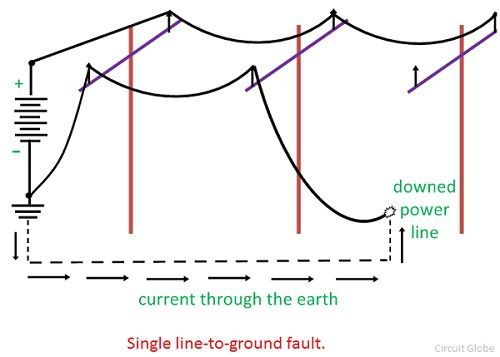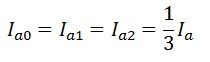# Single Line-to-Ground Fault

Generally, a single line-to-ground fault on a transmission line occurs when one conductor drops to the ground or comes in contact with the neutral conductor. Such types of failures may occur in power system due to many reasons like high-speed wind, falling off a tree, lightning, etc.

###Circuit diagram of single line-to-ground fault

Suppose the phase a is connected to ground at the fault point F as shown in a figure below. Ia, Ib and Ic are the current and Va, Vb and Vc are the voltage across the three phase line a, b and c respectively. The fault impedance of the line is Zf.Since only phase a is connected to ground at the fault, phase b and c are open circuited and carries no current; i.e fault current is Ia and Ib = 0, Ic = 0. The voltage at the fault point F is Va = Zf Ia.

The symmetrical component of the fault current in phase “a” at the fault point can be written asIn the case of a single line-to-ground fault, the sequence currents are equal. The sequence voltage at the fault point is determined by the equations:-Where, Ea0, Ea1, and Ea2 are the sequence voltages of phase a, and Za0, Za1 and Za2 are the sequence impedances to the flow of currents Ia0, Ia1, and Ia2 respectively. For a balanced systemOn substituting the Ia0 = Ia1 = Ia2 = Ia in above equation we get,The sequence current is given by equation,